## Sample Test Problems

1. Two identical spin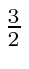particles are bound together into a state with total angular momentum. a) What are the allowed states of total spin forand for? b) List the allowed states using spectroscopic notation forand 1.2. A hydrogen atom is in the state. A combined measurement of of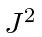and ofis made. What are the possible outcomes of this combined measurement and what are the probabilities of each? You may ignore nuclear spin in this problem.

3. We want to find the eigenstates of total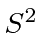andfor two spin 1 particles which have aninteraction. ()

1. What are the allowed values of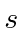, the total spin quantum number.
2. Write down the states of maximumfor the maximumstate. Usenotation andfor the product states.
3. Now apply the lowering operator to get the otherstates. You only need to go down tobecause of the obvious symmetry.
4. Now find the states with the other values ofin a similar way.

4. Two (identical) electrons are bound in a Helium atom. What are the allowed statesif both electrons have principal quantum number? What are the states if one hasand the other?

5. A hydrogen atom is in an eigenstateof,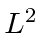, and ofsuch that,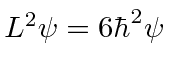,, and of course the electron's spin is. Determine the quantum numbers of this state as well as you can. If a measurement ofis made, what are the possible outcomes and what are the probabilities of each.

6. A hydrogen atom is in the state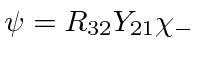. If a measurement ofand ofis made, what are the possible outcomes of this measurement and what are the probabilities for each outcome? If a measurement of the energy of the state is made, what are the possible energies and the probabilities of each? You may ignore the nuclear spin in this problem.

7. Two identical spin 1 particles are bound together into a state with orbital angular momentum. What are the allowed states of total spin (s) for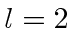, for, and for? List all the allowed states giving, for each state, the values of the quantum numbers for total angular momentum, orbital angular momentumand spin angular momentum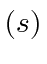ifis 2 or less. You need not list all the differentvalues.

8. List all the allowed states of total spin and total z-component of spin for 2 identical spin 1 particles. Whatvalues are allowed for each of these states? Explicitly write down the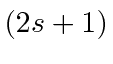states for the highestin terms of, and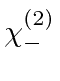.

9. Two different spinparticles have a Hamiltonian given by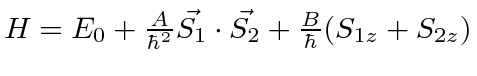. Find the allowed energies and the energy eigenstates in terms of the four basis states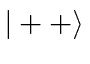,,, and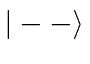.

10. A spin 1 particle is in anstate. Find the allowed values of the total angular momentum quantum number,. Write out thestates for the largest allowedvalue, in terms of thebasis. (That is give one state for everyvalue.) If the particle is prepared in the state, what is the probability to measure?

11. Two different spinparticles have a Hamiltonian given by. Find the allowed energies and the energy eigenstates in terms of the four product states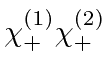,,, and.

Jim Branson 2013-04-22